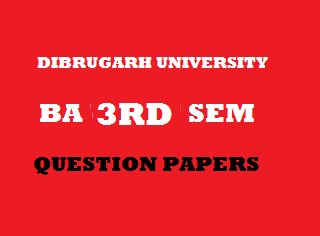## Friday, January 04, 20192013
(November)
MATHEMATICS
(Major)
Course: 301
[Analysis-I (Real Analysis)]
Full Marks: 80
Pass Marks: 32
Time: 3 hours
The figures in the margin indicate full marks for the questions
GROUP – A
(Differential Calculus)
(Marks: 35)

1. (a) Ifthen write the value ofis the positive integer. 1
(b) Find the value of 2(c) Show that the subnormal at any point of a parabola is of constant length. 3
Or
Find the curvature of the ellipseandat(d) Ifthen show that 4Or
Ifis the angle between the radius vector and the tangent at any point of the curvethen prove that2. (a) State Darboux’s theorem in differential calculus. 1
(b) If a functionis continuous inand differentiable insuch thatthen prove thatis strictly increasing on. 2
(c) State and prove Cauchy’s mean value theorem. 3
Of
Ifis continuous onand differentiable inandthen show that(d) Prove that4
Or
Expandin powers offor3. (a) State and Euler’s theorem on homogeneous function of two variables. 1
(b) Ifthen prove that4
Or
If, then prove that4. (a) State Schwarz’s theorem. 1
(b) Ifthen show that 2(c) Ifthen prove that3
(d) Prove thathas neither maximum nor minimum at4
Or
If, where, then prove thatGROUP – B
(Integral Calculus)
(Marks: 20)

5. (a) Ifthen write the relation betweenand1
(b) Show that2
(c) Evaluate:3
(d) Deduce the reduction formula for4
Or
Prove that6. (a) Ifthen write the length of arcwhere A and B have abscissa a and b respectively. 1
(b) Find the whole length of the curve5
Or
Find the volume of the solid generated by revolving the ellipseabout the major axis.
(c) Find the surface of the solid formed by revolving the cardioidsabout the initial line.    4
GROUP – C
(Riemann Integral)
(Marks: 25)

7. (a) What is the essential condition for a functionto be Riemann integrable in [a, b]? 1
(b) Prove that for any two partitionsandof. 2
(c) Show that every monotonic function onis Riemann integrable. 5
Or
Ifandare lower and upper bounds ofon, then show that8. (a) State first mean value theorem of integral calculus. 1
(b) A function having primitive may not be continuous. Justify it. 3
(c) Ifand g are integrable onandkeeps the same sign over, then show thatandwhere m, M are bounds of g on. 3
9. (a) Define Abel’s test for convergence of integral of a product. 1
(b) Show thatConverges to 0 2
(c) Test for convergence (any one): 3
1.2.10. (a) Prove that 4(b) Prove that***